Function Repository Resource:

# NthGrayCodeSubset

Get the n-th Gray code subset of a list

Contributed by: Wolfram Staff (original content by Sriram V. Pemmaraju and Steven S. Skiena)
 ResourceFunction["NthGrayCodeSubset"][n,list] gives the nth subset of list, listed in Gray code order.

## Details and Options

In Gray code order, subsets differ from its neighbors by the insertion or deletion of one element.
In ResourceFunction["NthGrayCodeSubset"][], the empty set is indexed as set 0.
ResourceFunction["NthGrayCodeSubset"] extracts parts cyclically from a List of all possible subsets.

## Examples

### Basic Examples (1)

Fifth subset of a List in Gray code order:

 In:=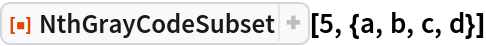Out=### Properties and Relations (2)

In Gray code order, subsets differ from their neighbors by by the insertion or deletion of exactly one element:

 In:=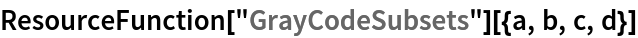Out=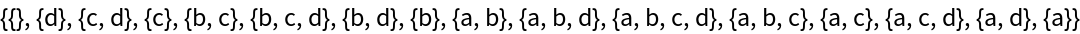Sequentially reconstruct the subset list with NthGrayCodeSubset:

 In:=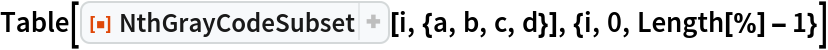Out=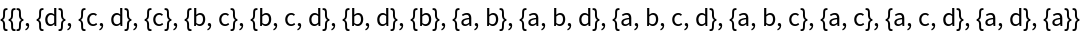In:=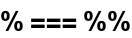Out=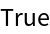The resource function GrayCodeSubsetIndex gives back the index n of the subset constructed with NthGrayCodeSubset:

 In:=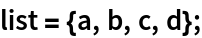In:=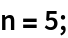In:=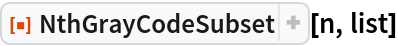Out=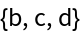In:=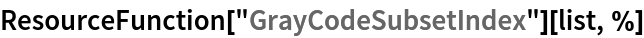Out=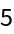## Version History

• 1.0.0 – 24 July 2020